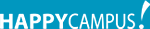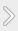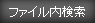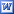#### タグ

レポート理工学角度変換行列

### 資料紹介

A rectangle OABC rotates counter clockwise about the point O as shown in the diagram below, which is not drawn to scale

Initially O is at (0,0), A is at (4,0), B is at (4,3), C is at (0,4).

The diagram shows the rectangle after it has rotated through 20 degrees. The vertices are now labeled O, A1, B1 and C1.

### 資料の原本内容( この資料を購入すると、テキストデータがみえます。 )

1. Rotating Rectangles A rectangle OABC rotates counter clockwise about the point O as shown in the diagram below, which is not drawn to scale
Initially O is at (0,0), A is at (4,0), B is at (4,3), C is at (0,4).
The diagram shows the rectangle after it has rotated through 20 degrees. The vertices are now labeled O, A1, B1 and C1.
1. Calculate the coordinates of A1 and C1. l A l = l A1 l thus We can make next triangle.
Now, we can use a sine rule.
O D becomes the A1's x-coordinate and
A1D becom..

## featherの資料(176)

•Relax
•Report image
•Simple and Stylish
•Stylish green
•Vision

### コメント追加

コメントを書込むには会員登録するか、すでに会員の方はログインしてください。